# Create Bell Curve Chart

The Bell curve chart is also called as normal probability distributions in Statistics and it will be used to show a plot of normal distribution of a given data set. This post will guide you how to create a chart of a bell curve in Microsoft Excel. And How to save the generated bell curve chart as normal chart template in Excel.

## Create Bell Curve Chart

You can refer to the below examples to create a sample bell curve, just do the following steps:

#1 enter the following column headings in a new worksheet (Data, Mean, Standard deviation, Distribution)

The mean is the average value of all the data points in range A2:A10. And the Standard Deviation indicates how much deviates from the mean of the data set.

#2 enter the data in the range A2:A10

#3 enter the formula =AVERAGE(A2:A10) in the formula box of cell B2 to generate the Mean value.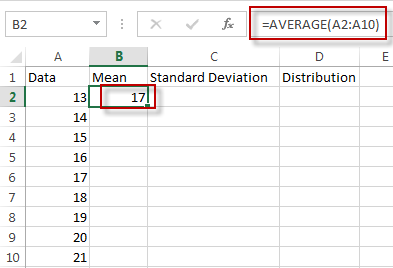#4 enter the formula =STDEV(A2:A10) in the formula box of cell C2 to generate the standard deviation.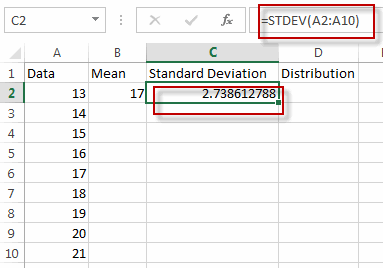#5 enter the formula =NORM.DIST(A2,\$B\$2,\$C\$2,FALSE) in the formula box of cell D2 to generate normal probability distribution. Then drag Auto Fill Handler over other cells from D2 to D10.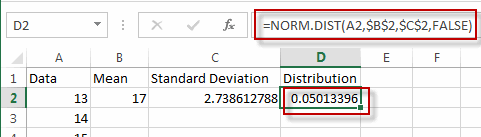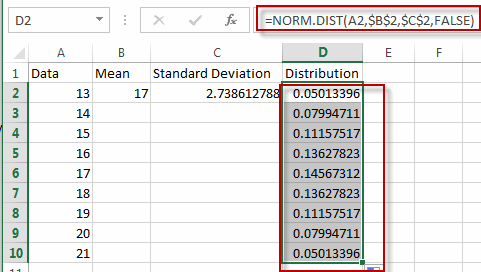#6 select range A2:A10 and D2:D10, then go to INSERT tab, click Scatter with Smooth Lines under charts group.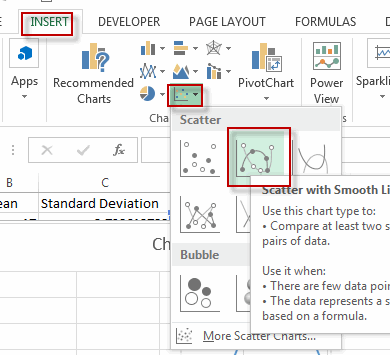#7 you will see a bell curve is created.

create bell curve chart5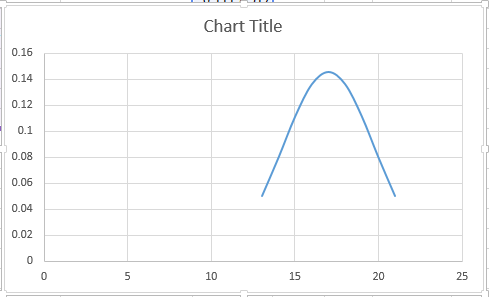## Save Bell Curve Chart as Chart Template

If you want to save the generated bell curve chart as chart template, just refer to the below steps:

#1 right click on the bell curve chart, select Save as Template… from the pop up menu list.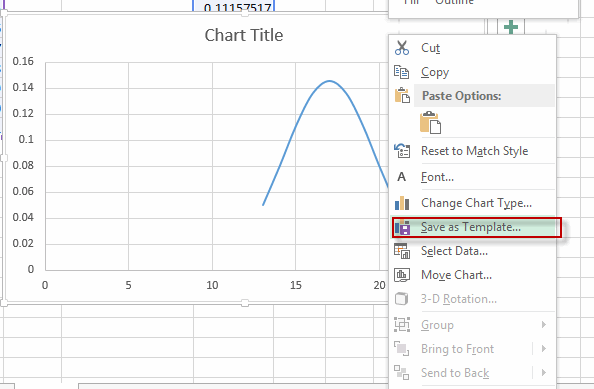#2 enter the file name, and click Save button.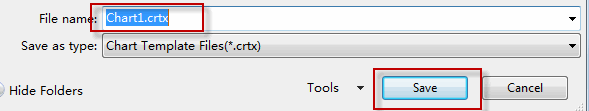### Related Functions

• Excel AVERAGE function
The Excel AVERAGE function returns the average of the numbers that you provided.The syntax of the AVERAGE function is as below:=AVERAGE (number1,[number2],…)….
• Excel STDEV Function
The Excel STDEV function returns the standard deviation of a population based on a sample of numbers. This function has been replaced by the STDEV.S function in Excel 2010.The syntax of the STDEV function is as below:= STDEV (number1,[number2],…)…